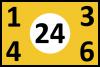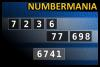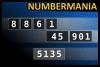BRAIN TEASERS
Brain Teasers User Profile

# llewellyn samuels

rank
57
points
438
See full ranking list
short ranking list
 55 Ranga Nathan 480 56 Rafael Rios 447 57 llewellyn samuels 438 58 Llewellyn Samuels 438 59 Adman72 Rocks 388
 Calculate the number 24 Calculate the number 24 using numbers [1, 3, 4, 6] and basic arithmetic operations (+, -, *, /). Each of the numbers must be used only once.Calculate the number 6741 NUMBERMANIA: Calculate the number 6741 using numbers [7, 2, 3, 6, 77, 698] and basic arithmetic operations (+, -, *, /). Each of the numbers can be used only once.Calculate the number 5135 NUMBERMANIA: Calculate the number 5135 using numbers [8, 8, 6, 1, 45, 901] and basic arithmetic operations (+, -, *, /). Each of the numbers can be used only once.